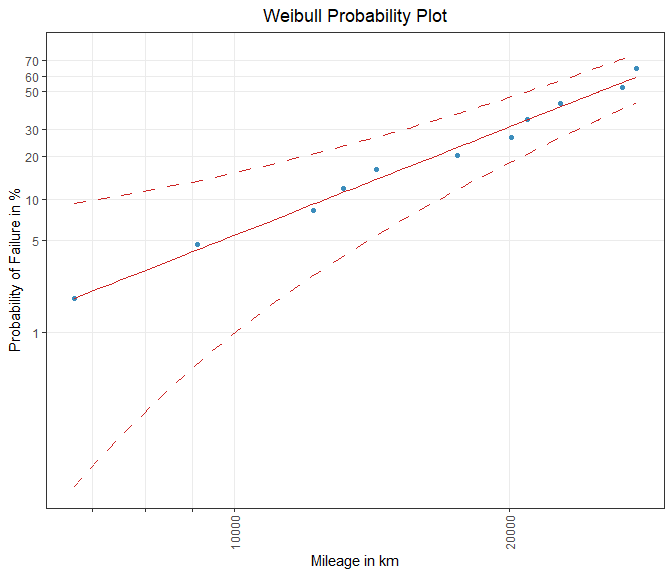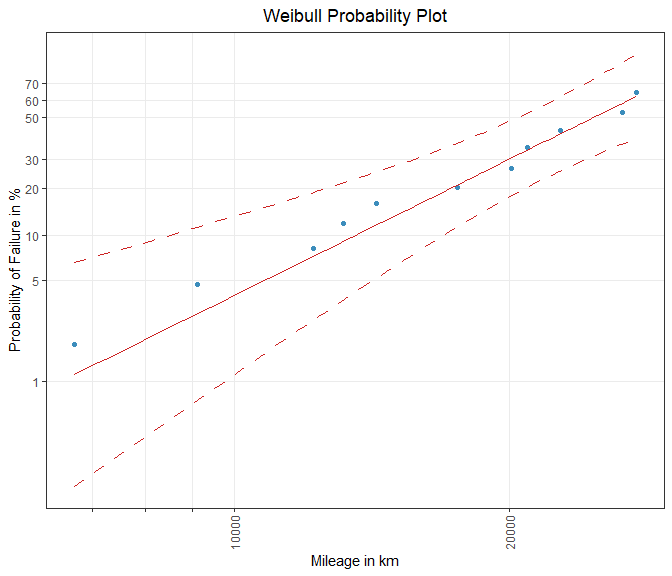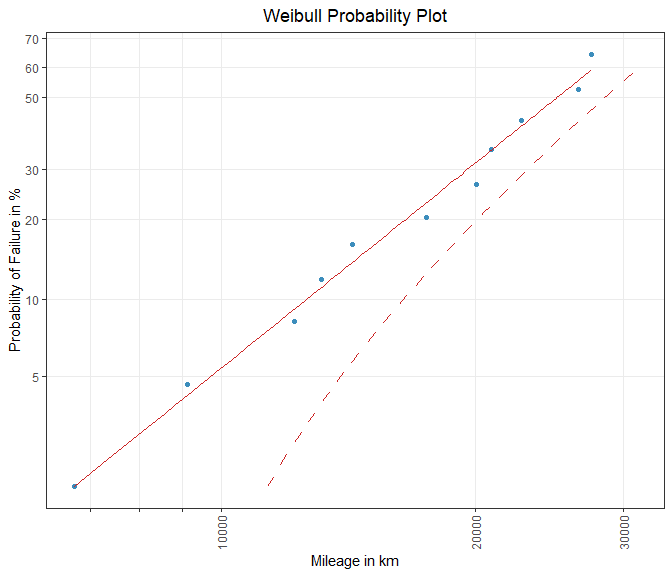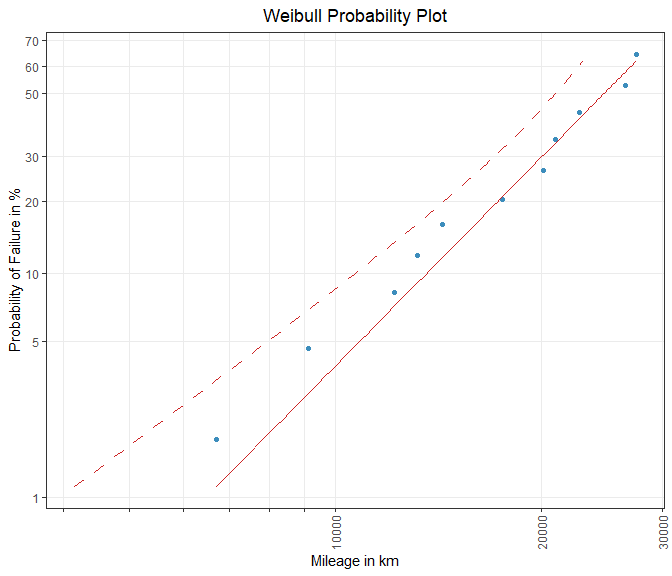# Life Data Analysis Part III - Confidence Intervals

### For Model Parameters, Probabilities and Quantiles

#### 2023-04-04

In contrast to point estimation procedures, interval estimation methods, e.g. the computation of confidence intervals, express the uncertainty which is associated with the use of a statistical estimator.

In reliability analysis it is common practice to provide confidence regions for the parameters of a lifetime distribution as well as for quantities that depend on these model parameters.

In this vignette, the determination of confidence intervals for model parameters, quantiles (lifetime characteristic) and failure probabilites (CDF) is presented.

## Confidence Intervals for Model Parameters

Confidence intervals can be calculated for every model parameter. For this, the (approximated) sampling distribution as well as an estimate of its standard deviation must be given. In the following, the formulas which strongly depend on the estimation methods, are provided for Rank Regression and Maximum Likelihood Estimation.

### Rank Regression (RR)

In Rank Regression a linear relationship between the lifetime characteristic and the failure probability is determined. The parameters of a simple linear regression model are the intercept and the slope, which are the location parameter $$\mu$$ and the scale parameter $$\sigma$$ for the majority of lifetime distributions.

An approximated two-sided interval for the true parameters on a $$1 - \alpha$$ confidence level can be obtained with the formulas

$\bigg[\hat{\mu}_{\text{lower}} \, ; \hat{\mu}_{\text{upper}} \bigg] = \bigg[\hat{\mu}_{\text{RR}} \pm t_{1 - \frac{\alpha}{2}} \cdot \hat{se}^{\text{HC}}_{\hat{\mu}}\bigg] \qquad and \qquad \bigg[\hat{\sigma}_{\text{lower}} \, ;\hat{\sigma}_{\text{upper}} \bigg] = \bigg[\hat{\sigma}_{\text{RR}} \pm t_{1 - \frac{\alpha}{2}} \cdot \hat{se}^{\text{HC}}_{\hat{\sigma}}\bigg].$

For a given sample, $$\hat{\mu}_{\text{RR}}$$ and $$\hat{\sigma}_{\text{RR}}$$ are the least squares estimates and $$\hat{se}^{\text{HC}}_{\hat{\mu}}$$ and $$\hat{se}^{\text{HC}}_{\hat{\sigma}}$$ are the respective estimates of the standard deviations. The uncertainty that arises due to the unknown standard deviations must be taken into account by using the quantiles of Student’s t-distribution.

When using RR in the context of reliability analysis, the assumption of homoscedastic error terms is often violated. Therefore, the computation of the standard errors is based on a heteroscedasticity-consistent (HC) variance-covariance matrix. Other assumptions of the classical linear regression model like the need of no serial correlation are questionable as well and have already been discussed in the literature 1.

Hence, the Maximum Likelihood Estimation procedure is recommended, which is described in the next section.

### Maximum Likelihood Estimation (MLE)

ML estimators are subject to a variety of restrictions but in return have many useful properties in contrast to other estimation techniques. One is that ML estimators converge in distribution to a normal distribution and for this reason, normal approximation confidence intervals for the model parameters can be calculated by theory.

Using the parameterization introduced above, a two-sided normal approximation confidence interval for the location parameter $$\mu$$ can be computed with the equation

$\bigg[\hat{\mu}_{\text{lower}} \, ; \, \hat{\mu}_{\text{upper}} \bigg] = \bigg[\hat{\mu}_{\text{MLE}} \pm z_{1 - \frac{\alpha}{2}} \cdot \hat{se}_{\hat{\mu}}\bigg].$

By definition, the scale parameter $$\sigma$$ is always positive and thus an alternative confidence interval is used 2:

$\bigg[\hat{\sigma}_{\text{lower}} \, ; \, \hat{\sigma}_{\text{upper}} \bigg] = \bigg[\frac{\hat{\sigma}_{\text{MLE}}}{w} \, ; \hat{\sigma}_{\text{MLE}} \cdot w \bigg]$ with

$w = \exp\left[z_{1 - \frac{\alpha}{2}} \cdot \frac{\hat{se}_{\hat{\sigma}}}{\hat{\sigma}_{\text{MLE}}}\right].$

## Confidence Intervals for Probabilities and Quantiles

In addition to the confidence regions for the distribution-specific parameters, intervals for the regression line are provided as well. These can be aligned according to the probability $$F(t)$$ or to the lifetime characteristic $$t$$.

Whereas the Beta-Binomial confidence bounds are often used in combination with RR, Fisher’s normal approximation confidence intervals are only applicable in the case of MLE.

### Beta-Binomial Confidence Intervals for $$F(t)$$

To obtain a two-sided non-parametric confidence interval for the failure probabilities at a given $$1-\alpha$$ level, a procedure similar to Median Ranks (MR) is used.

Instead of finding the probability $$p_{\text{MR}}$$ for the j-th rank at the $$50\%$$ level

$0.5 = \sum^n_{k = j} \binom{n}{k} \cdot p_{\text{MR}}^k \cdot \left(1-p_{\text{MR}}\right)^{n-k},$

the probability $$p_{\text{lower}}$$ must be determined for equation

$\frac{\alpha}{2} = \sum^n_{k = j} \binom{n}{k} \cdot p_{\text{lower}}^k \cdot \left(1-p_{\text{lower}}\right)^{n-k}$

and $$p_{\text{upper}}$$ for the expression

$1 - \frac{\alpha}{2} = \sum^n_{k = j} \binom{n}{k} \cdot p_{\text{upper}}^k \cdot \left(1-p_{\text{upper}}\right)^{n-k}.$

The resulting interval $$\left[\hat{F}_{j, \, {\text{lower}}} \, ; \, \hat{F}_{j, \, {\text{upper}}}\right] = \left[\hat{p}_{{\text{lower}}} \, ; \, \hat{p}_{{\text{upper}}}\right]$$ is the estimated confidence region for the true failure probability with respect to the j-th rank.

### Beta-Binomial Confidence Intervals for $$t$$

Once the intervals of the failure probabilities are calculated, a two-sided confidence interval for the lifetime characteristic can be found with the quantile function of the underlying lifetime distribution.

For the Weibull, the quantile function is given by the formula

$t_{p} = F^{-1}(p) = \exp\left[\mu + \Phi^{-1}_{\text{SEV}}(p) \cdot \sigma\right],$ where $$\Phi^{-1}_{\text{SEV}}$$ is the quantile function of the standard smallest extreme value distribution.

The confidence interval for $$t$$ with respect to the estimated RR parameters as well as the lower and upper probability of the j-th rank is then computed by

$\hat{t}_{j \, ; \, \text{lower}} = \exp\left[\hat{\mu}_{\text{RR}} + \Phi^{-1}_{\text{SEV}}(\hat{F}_{j, \, {\text{lower}}}) \cdot \hat{\sigma}_{\text{RR}}\right]$ and

$\hat{t}_{j \, ; \, \text{upper}} = \exp\left[\hat{\mu}_{\text{RR}} + \Phi^{-1}_{\text{SEV}}(\hat{F}_{j, \, {\text{upper}}}) \cdot \hat{\sigma}_{\text{RR}}\right].$

### Fisher’s Confidence Intervals for $$F(t)$$

For a particular quantile $$t$$ and the vector of parameters $$\hat{\theta}_{MLE}$$, a normal approximation confidence interval for the failure probability $$F(t)$$ can be obtained by

$\bigg[\hat{F}_{\text{lower}}(t) \, ; \, \hat{F}_{\text{upper}}(t)\bigg] = \bigg[\hat{F}_{\text{MLE}}(t) \pm z_{1 - \frac{\alpha}{2}} \cdot \hat{se}_{\hat{F}(t)}\bigg].$

In order to guarantee that the realized confidence interval of $$F(t)$$ always is between 0 and 1, the so called z-procedure can be applied 3. Using this technique, statistical inference is first done for the standardized quantile $$z$$ and afterwards entered in $$F(t)$$ to obtain the desired interval.

For the Weibull, the ML estimator of the standardized quantile function $$z$$ is

$\hat{z}_{\text{MLE}} = \frac{log(t) - \hat{\mu}_{\text{MLE}}}{\hat{\sigma}_{\text{MLE}}}.$

First, an approximate confidence interval for $$z$$ is determined with the following formula:

$\bigg[\hat{z}_{\text{lower}} \, ; \, \hat{z}_{\text{upper}}\bigg] = \bigg[\hat{z}_{\text{MLE}} \pm z_{1 - \frac{\alpha}{2}} \cdot \hat{se}_{\hat{z}}\bigg].$

An approximate formula for the standard error of the estimator $$\hat{z}$$ can be derived with the delta method:

$\hat{se}_{\hat{z}} = \sqrt{\hat{Var}_{\hat{z}}} = \sqrt{\bigg(\frac{\partial{\hat{z}}}{\partial{\hat{\theta}_{\text{MLE}}}}\bigg)^{T}\; \hat{Var}(\hat{\theta}_{\text{MLE}})\; \frac{\partial{\hat{z}}}{\partial{\hat{\theta}_{\text{MLE}}}}} \; .$

Finally, the estimated bounds of $$z$$ are then plugged into the distribution-specific standard CDF to obtain the interval for $$F(t)$$, which is

$\bigg[\hat{F}_{\text{lower}}(t) \, ; \, \hat{F}_{\text{upper}}(t)\bigg] = \bigg[\Phi_{\text{SEV}}(\hat{z}_{\text{lower}}) \, ; \, \Phi_{\text{SEV}}(\hat{z}_{\text{upper}}) \bigg].$

### Fisher’s Confidence Intervals for $$t$$

In reliability analysis the lifetime characteristic often is defined as a strictly positive quantity and hence, a normal approximation confidence interval for the quantile $$t$$ with respect to a particular probability $$p$$ and the vector of parameters $$\hat{\theta}_{MLE}$$, can be calculated by

$\bigg[\hat{t}_{\text{lower}}(p) \, ; \, \hat{t}_{\text{upper}}(p)\bigg] = \bigg[\frac{\hat{t}_{\text{MLE}}(p)}{w} \, ; \hat{t}_{\text{MLE}}(p) \cdot w \bigg],$ where $$w$$ is

$w = \exp\left[z_{1 - \frac{\alpha}{2}} \cdot \frac{\hat{se}_{\hat{t}(p)}}{\hat{t}_{\text{MLE}}(p)}\right].$

For the Weibull, the ML equation for the quantile $$t(p)$$ is

$\hat{t}_{\text{MLE}}(p) = \exp\left[\hat{\mu}_{\text{MLE}} + \Phi^{-1}_{\text{SEV}}(p) \cdot \hat{\sigma}_{\text{MLE}}\right]$

and again, through the use of the delta method, a formula for the standard error of $$\hat{t}_p$$ can be provided, which is

$\hat{se}_{\hat{t}(p)} = \sqrt{\hat{Var}_{\hat{t}(p)}} = \sqrt{\bigg(\frac{\partial{\hat{t}(p)}}{\partial{\hat{\theta}_{\text{MLE}}}}\bigg)^{T}\; \hat{Var}(\hat{\theta}_{\text{MLE}})\; \frac{\partial{\hat{t}(p)}}{\partial{\hat{\theta}_{\text{MLE}}}}} \; .$

## Data

For the computation of the presented confidence intervals the shock dataset is used. In this dataset kilometer-dependent problems that have occurred on shock absorbers are reported. In addition to failed items the dataset also contains non-defective (censored) observations. The data can be found in Statistical Methods for Reliability Data 4.

For consistent handling of the data, {weibulltools} introduces the function reliability_data() that converts the original dataset into a wt_reliability_data object. This formatted object allows to easily apply the presented methods.

# Data:
shock_tbl <- reliability_data(data = shock, x = distance, status = status)
shock_tbl
#> Reliability Data with characteristic x: 'distance':
#> # A tibble: 38 × 3
#>        x status id
#>    <int>  <dbl> <chr>
#>  1  6700      1 ID1
#>  2  6950      0 ID2
#>  3  7820      0 ID3
#>  4  8790      0 ID4
#>  5  9120      1 ID5
#>  6  9660      0 ID6
#>  7  9820      0 ID7
#>  8 11310      0 ID8
#>  9 11690      0 ID9
#> 10 11850      0 ID10
#> # ℹ 28 more rows

## Confidence Intervals with {weibulltools}

Before calculating confidence intervals with {weibulltools} one has to conduct the basic steps of the Weibull analysis which are described in the previous vignettes.

# Estimation of failure probabilities:
shock_cdf <- estimate_cdf(shock_tbl, methods = "johnson")

# Rank Regression:
rr_weibull <- rank_regression(shock_cdf, distribution = "weibull")

# Maximum Likelihood Estimation:
ml_weibull <- ml_estimation(shock_tbl, distribution = "weibull")

### Confidence Intervals for Model Parameters

The confidence intervals for the distribution parameters are included in the model output of rank_regression() and ml_estimation(), respectively.

# Confidence intervals based on Rank Regression:
rr_weibull$confint #> 2.5 % 97.5 % #> mu 10.2079027 10.3112576 #> sigma 0.3428984 0.3835118 # Confidence intervals based on Maximum Likelihood Estimation: ml_weibull$confint
#>            2.5 %     97.5 %
#> mu    10.0144824 10.4452437
#> sigma  0.2011036  0.4978267

The confint element of the model output is a matrix with the parameter names as row names and the confidence level as column names. Different levels can be specified using the argument conf_level.

# Confidence intervals based on another confidence level:
ml_weibull_99 <- ml_estimation(shock_tbl, distribution = "weibull", conf_level = 0.99)
ml_weibull_99\$confint
#>           0.5 %     99.5 %
#> mu    9.9468049 10.5129212
#> sigma 0.1744101  0.5740192

### Confidence Intervals for Probabilities

Confidence bounds for failure probabilities can be either determined with confint_betabinom() or confint_fisher(). As explained in the theoretical part of this vignette the Beta-Binomial confidence bounds should be applied to the output of rank_regression() whereas Fisher’s normal approximation confidence intervals are only applicable if the parameters and the variance-covariance matrix were estimated with ml_estimation().

# Beta-Binomial confidence bounds:
conf_bb <- confint_betabinom(
x = rr_weibull,
b_lives = c(0.01, 0.1, 0.5),
bounds = "two_sided",
conf_level = 0.95,
direction = "y"
)
conf_bb
#> # A tibble: 102 × 6
#>        x  rank   prob lower_bound upper_bound cdf_estimation_method
#>  * <dbl> <dbl>  <dbl>       <dbl>       <dbl> <chr>
#>  1 6700.  1.00 0.0183    0.000674      0.0927 johnson
#>  2 6910   1.06 0.0199    0.000859      0.0958 johnson
#>  3 7120.  1.13 0.0216    0.00108       0.0990 johnson
#>  4 7330.  1.20 0.0234    0.00134       0.102  johnson
#>  5 7540   1.27 0.0252    0.00165       0.106  johnson
#>  6 7750   1.34 0.0272    0.00201       0.109  johnson
#>  7 7960.  1.42 0.0293    0.00242       0.113  johnson
#>  8 8170   1.51 0.0314    0.00289       0.117  johnson
#>  9 8380   1.59 0.0336    0.00341       0.121  johnson
#> 10 8590   1.68 0.0360    0.00401       0.124  johnson
#> # ℹ 92 more rows

# Fisher's normal approximation confidence intervals:
conf_fisher <- confint_fisher(x = ml_weibull)
conf_fisher
#> # A tibble: 102 × 6
#>        x   prob std_err lower_bound upper_bound cdf_estimation_method
#>  * <dbl>  <dbl>   <dbl>       <dbl>       <dbl> <chr>
#>  1 6700. 0.0112   0.916     0.00186      0.0655 <NA>
#>  2 6910  0.0123   0.895     0.00214      0.0691 <NA>
#>  3 7120. 0.0135   0.875     0.00245      0.0729 <NA>
#>  4 7330. 0.0148   0.855     0.00279      0.0767 <NA>
#>  5 7540  0.0162   0.835     0.00317      0.0805 <NA>
#>  6 7750  0.0177   0.817     0.00359      0.0845 <NA>
#>  7 7960. 0.0192   0.799     0.00404      0.0886 <NA>
#>  8 8170  0.0208   0.781     0.00454      0.0927 <NA>
#>  9 8380  0.0225   0.764     0.00509      0.0969 <NA>
#> 10 8590  0.0244   0.747     0.00568      0.101  <NA>
#> # ℹ 92 more rows

The outputs of both functions contain the calculated bounds for the failure probabilities ranging from the minimum to the maximum observed failure. Between the observed range of failures an interpolation of quantiles is made for which the intervals of the probabilities are provided as well (supporting points).

In the function call of confint_betabinom() the default arguments of both functions are listed. With the argument b_lives, confidence regions for selected probabilities are included, but only if they are in the range of the estimated failure probabilities.

The argument bounds is used for the specification of the bound(s) to be computed. It could be one of c("two_sided", "lower", "upper").

If direction = "y", confidence intervals for the probabilities are provided.

The visualization of the computed intervals is done with plot_conf().

# Probability plot
weibull_grid <- plot_prob(
shock_cdf,
distribution = "weibull",
title_main = "Weibull Probability Plot",
title_x = "Mileage in km",
title_y = "Probability of Failure in %",
title_trace = "Defectives",
plot_method = "ggplot2"
)
# Beta-Binomial confidence intervals:
weibull_conf_bb <- plot_conf(
weibull_grid,
conf_bb,
title_trace_mod = "Rank Regression",
title_trace_conf = "Beta-Binomial Bounds"
)
weibull_conf_bbFigure 1: Beta-Binomial confidence bounds for failure probabilities.

# Fisher's normal approximation confidence intervals:
weibull_conf_fisher <- plot_conf(
weibull_grid,
conf_fisher,
title_trace_mod = "Maximum Likelihood",
title_trace_conf = "Fisher's Confidence Intervals"
)
weibull_conf_fisherFigure 2: Fisher’s normal approximation confidence intervals for failure probabilities.

As one can see, plot_conf() not only adds the confidence limits to an existing probability plot, but also includes the estimated linearized CDF. There is no need for an additional call of plot_mod(). In fact, the same routines used by plot_mod() are called under the hood, which ensures that confidence bounds are not drawn without the regression line.

### Confidence Intervals for Quantiles

The computation and visualization of confidence for the lifetime characteristic is pretty similar to the presented procedure with regard to the probabilities. The only difference is that one has to change the value of the argument direction to "x".

# Computation of confidence intervals for quantiles:
## Beta-Binomial confidence intervals:
conf_bb_x <- confint_betabinom(
x = rr_weibull,
bounds = "upper",
conf_level = 0.95,
direction = "x"
)
conf_bb_x
#> # A tibble: 102 × 5
#>        x  rank   prob upper_bound cdf_estimation_method
#>  * <dbl> <dbl>  <dbl>       <dbl> <chr>
#>  1 6700.  1.00 0.0183      11357. johnson
#>  2 6910   1.06 0.0199      11521. johnson
#>  3 7120.  1.13 0.0216      11687. johnson
#>  4 7330.  1.20 0.0234      11855. johnson
#>  5 7540   1.27 0.0252      12024. johnson
#>  6 7750   1.34 0.0272      12195. johnson
#>  7 7960.  1.42 0.0293      12367. johnson
#>  8 8170   1.51 0.0314      12541. johnson
#>  9 8380   1.59 0.0336      12715. johnson
#> 10 8590   1.68 0.0360      12891. johnson
#> # ℹ 92 more rows

## Fisher's normal approximation confidence intervals:
conf_fisher_x <- confint_fisher(x = ml_weibull, bounds = "lower", direction = "x")
conf_fisher_x
#> # A tibble: 102 × 5
#>        x   prob std_err lower_bound cdf_estimation_method
#>  * <dbl>  <dbl>   <dbl>       <dbl> <chr>
#>  1 6700. 0.0112   1943.       4159. <NA>
#>  2 6910  0.0123   1957.       4337. <NA>
#>  3 7120. 0.0135   1970.       4517. <NA>
#>  4 7330. 0.0148   1982.       4698. <NA>
#>  5 7540  0.0162   1993.       4881. <NA>
#>  6 7750  0.0177   2003.       5067. <NA>
#>  7 7960. 0.0192   2011.       5253. <NA>
#>  8 8170  0.0208   2019.       5441. <NA>
#>  9 8380  0.0225   2025.       5631. <NA>
#> 10 8590  0.0244   2031.       5822. <NA>
#> # ℹ 92 more rows
# Visualization:
## Beta-Binomial confidence intervals:
weibull_conf_bb_x <- plot_conf(
weibull_grid,
conf_bb_x,
title_trace_mod = "Rank Regression",
title_trace_conf = "Beta-Binomial Bounds"
)
weibull_conf_bb_xFigure 3: One-sided (upper) Beta-Binomial confidence bound for quantiles.

## Fisher's normal approximation confidence intervals:
weibull_conf_fisher_x <- plot_conf(
weibull_grid,
conf_fisher_x,
title_trace_mod = "Maximum Likelihood",
title_trace_conf = "Fisher's Confidence Intervals"
)
weibull_conf_fisher_xFigure 4: One-sided (lower) normal approximation confidence interval for quantiles.

1. Genschel, U.; Meeker, W. Q.: A Comparison of Maximum Likelihood and Median-Rank Regression for Weibull Estimation, in: Quality Engineering 22 (4), DOI: 10.1080/08982112.2010.503447, 2010, pp. 236-255↩︎

2. Meeker, W. Q.; Escobar, L. A.: Statistical Methods for Reliability Data, New York, Wiley series in probability and statistics, 1998, p. 188↩︎

3. Hoang, Y.; Meeker, W. Q.; Escobar, L. A.: The Relationship Between Confidence Intervals for Failure Probabilities and Life Time Quantiles, in: _IEEE Transactions on Reliability 57, 2008, pp. 260-266↩︎

4. Meeker, W. Q.; Escobar, L. A.: Statistical Methods for Reliability Data, New York, Wiley series in probability and statistics, 1998, p. 630↩︎